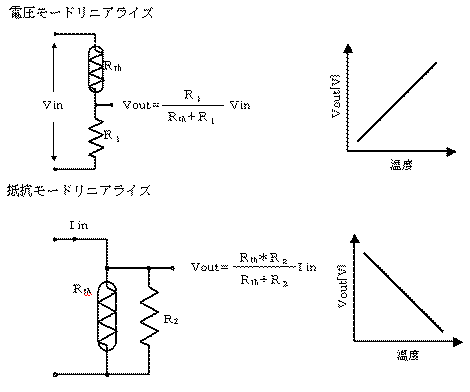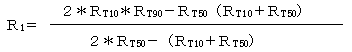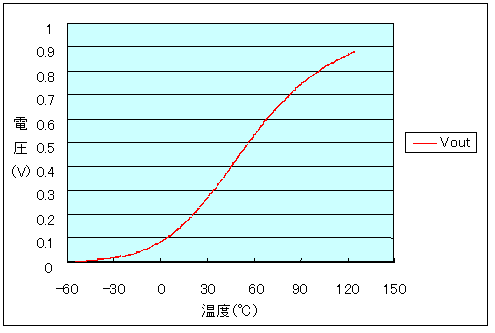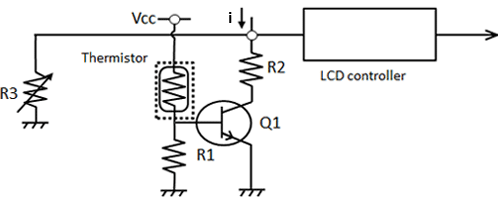# Products# Thermo-Sensitive Devices and Thermistors 2

## Thermistors in detail

A thermistor is a thermo-sensitive element which resistance value changes to the temperature. It has negative temperature coefficient (NTC), meaning its resistance decreases exponentially with increasing temperature. NT73 is lined up. The other type of thermistor with positive temperature coefficient (PTC), meaning its resistance increases exponentially with increasing temperature. These two types of thermistors are widely used in industry and consumer electronics. For example, PHS phone includes thermistors to control rechargeable batteries.

The characteristics of the negative temperature coefficient thermistor are given in the equation.

R=Ro×exp{B(1/T-1/To)}
where R and Ro are the resistance value at absolute temperature T(K) and To(K) respectively,
T(K)=t(℃)+273.15, and B is called as B constant whose dimension is (K).
Generally, Ro as of 25℃ is given in the catalogue.

## Linearization of the thermistor

The resistance of the thermistor varies exponentially with temperature as mentioned above, and the resistance changes very widely (i.e. more than 100 times) when the temperature ranges to some extent. Then it is convenient to narrow the variation for usage. This process is called the linearization, not in the strict sense but an approximation. There exists some standard circuits, involving one resistor type, or two or more resistors types.
One is a voltage mode linearizer circuit, where output voltage (Vout) increases with temperature. Another is a resistance mode linearizer circuit, where output voltage (Vout) decreases with temperature.A discussion on the voltage mode linearizer circuit is as follows:
Assume that the working temperature of the lower limit is TL and higher TH.
RT10 is the resistance value at the temperature higher by 10% of TL, RT50 and RT90 are higher by 50% and 90% respectively. Then the series resistance value R1 is calculated as;To give an numerical example, assuming that the device is used NT732ATD22k
The working temperature of lower limit: TL=0℃,
the working temperature of the upper limit: TH=100℃,
Ro(at 25℃)=22kΩ, B=3,800.
R=Ro exp { B ( 1/T-1/To) } …equation(1).
Substitution of their factors in this equation(1),
RT10, RT50, RT90 are 43.21, 8.21 and 2.25 kΩ respectively.
From these numerical values, R1 becomes 6.51kΩ.
Note from the plot, that Vout is well linearized between 0 and 100℃.## Thermistors to control the LCD

Thermistors are widely used in LCD panels of PHD's and various office equipment. The characteristics of LCD depend so much on ambient temperature that the contrast needs to be compensated by some thermo-sensitive device. A negative temperature coefficient thermistors is used to sense the temperature and control the voltage applied to the panel.

Compensation of LCD panel